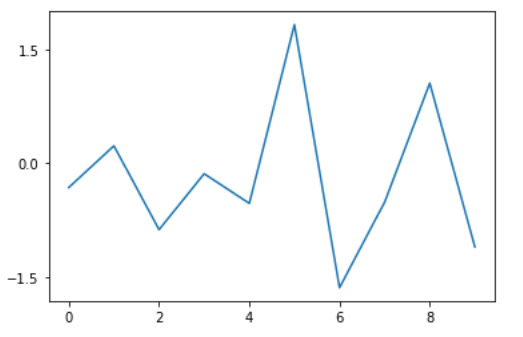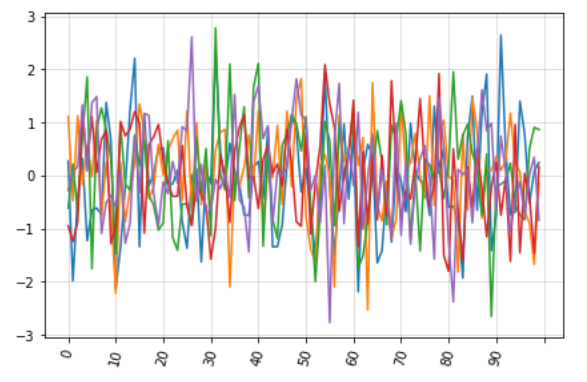Matplotlib.ticker.MaxNLocator Class in Python

• Last Updated : 21 Apr, 2020

Matplotlib is an amazing visualization library in Python for 2D plots of arrays. Matplotlib is a multi-platform data visualization library built on NumPy arrays and designed to work with the broader SciPy stack.

matplotlib.ticker.MaxNLocator

The matplotlib.ticker.MaxNLocator class is used to select no more than N intervals at nice locations. It is a subclass of matplotlib.ticker.Locator.

Syntax: class matplotlib.ticker.MaxNLocator(*args, **kwargs)

Parameter:

• nbins: It is either an integer or ‘auto’, where the integer value represents the maximum number of intervals; one less than max number of ticks. The number of bins gets automatically determined on the basis of the length of the axis.It is an optional argument and has a default value of 10.
• steps: It is an optional parameter representing a nice number sequence that starts from 1 and ends with 10.
• integer: It is an optional boolean value. If set True, the ticks accepts only integer values, provided at least min_n_ticks integers are within the view limits.
• symmetric: It is an optional value. If set to True, auto-scaling will result in a range symmetric about zero.
• prune: It is an optional parameter that accepts either of the four values: {‘lower’, ‘upper’, ‘both’, None}. By default it is None.

Methods of the class:

• set_params(self, **kwargs): It sets parameters for the locator.
• tick_values(self, vmin, vmax): It returns the values of the located ticks given vmin and vmax.
• view_limits(self, dmin, dmax): It is used to select a scale for the range from vmin to vmax.

Example 1:

 import matplotlib.pyplot as pltfrom matplotlib import tickerimport numpy as np    N = 10x = np.arange(N)y = np.random.randn(N)  fig = plt.figure()ax = fig.add_subplot(111)ax.plot(x, y)  # Create your ticker object with M ticksM = 3yticks = ticker.MaxNLocator(M)  # Set the yaxis major locator using# your ticker object. ax.yaxis.set_major_locator(yticks)  plt.show()

Output:Example 2:

 import matplotlib.pyplot as pltfrom matplotlib.ticker import MaxNLocator, IndexFormatter    ax = df.plot()  ax.xaxis.set_major_locator(MaxNLocator(11))ax.xaxis.set_major_formatter(IndexFormatter(df.index))   ax.grid(which ='minor', alpha = 0.2)ax.grid(which ='major', alpha = 0.5)  ax.legend().set_visible(False)plt.xticks(rotation = 75)plt.tight_layout()  plt.show()

Output:My Personal Notes arrow_drop_up